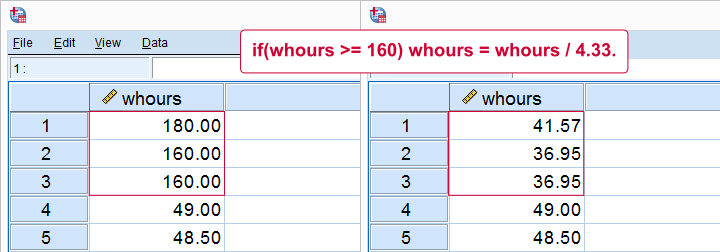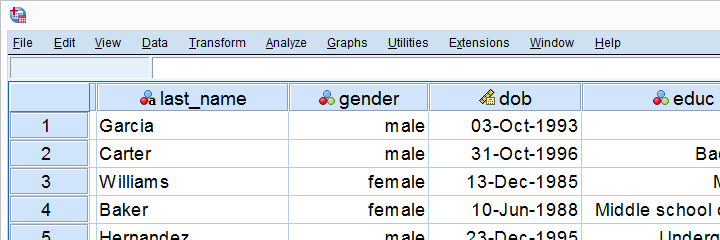# SPSS tutorials

BASICS DATA ANALYSIS T-TEST ANOVA CHI-SQUARE TEST

# SPSS IF – A Quick Tutorial

In SPSS, IF computes a new or existing variable
for a selection of cases.
For analyzing a selection of cases, use FILTER or SELECT IF instead.## Data File Used for Examples

All examples use bank.sav, a short survey of bank employees. Part of the data are shown below. For getting the most out of this tutorial, we recommend you download the file and try the examples for yourself.## Example 1 - Flag Cases Based on Date Function

Let's flag all respondents born during the 80’s. The syntax below first computes our flag variable -born80s- as a column of zeroes. We then set it to one if the year -extracted from the date of birth- is in the RANGE 1980 through 1989.

*Create new variable holding only zeroes.
compute born80s = 0.

*Set value to 1 if respondent born between 1980 and 1989.
if(range(xdate.year(dob),1980,1989)) born80s = 1.
execute.

add value labels born80s 0 'Not born during 80s' 1 'Born during 80s'.

## Result## Example 2 - Replace Range of Values by Function

Next, if we'd run a histogram on weekly working hours -whours- we'd see values of 160 hours and over. However, weeks only hold (24 * 7 =) 168 hours. Even Kim Jong Un wouldn't claim he works 160 hours per week!
We assume these respondents filled out their monthly -rather than weekly- working hours. On average, months hold (52 / 12 =) 4.33 weeks. So we'll divide weekly hours by 4.33 but only for cases scoring 160 or over.

*Sort cases descendingly on weekly hours.
sort cases by whours (d).

*Divide 160 or more hours by 4.33 (average weeks per month).
if(whours >= 160) whours = whours / 4.33.
execute.

## Result## Note

We could have done this correction with RECODE as well: RECODE whours (160 = 36.95)(180 = 41.57). Note, however, that RECODE becomes tedious insofar as we must correct more distinct values. It works reasonably for this variable but IF works great for all variables.

## Example 3 - Compute Variable Differently Based on Gender

We'll now flag cases who work fulltime. However, “fulltime” means 40 hours for male employees and 36 hours for female employees. So we need to use different formulas based on gender. The IF command below does just that.

*Compute fulltime holding only zeroes.
compute fulltime = 0.

*Set fulltime to 1 if whours >= 36 for females or whours >= 40 for males.
if(gender = 0 & whours >= 36) fulltime = 1.
if(gender = 1 & whours >= 40) fulltime = 1.

add value labels fulltime 0 'Not working fulltime' 1 'Working fulltime'.

*Quick check.
means whours by gender by fulltime
/cells min max mean stddev.

## Result

Our syntax ends with a MEANS table showing minima, maxima, means and standard deviations per gender per group. This table -shown below- is a nice way to check the results.The maximum for females not working fulltime is below 36. The minimum for females working fulltime is 36. And so on.

## SPSS IF Versus DO IF

Some SPSS users may be familiar with DO IF. The main differences between DO IF and IF are that

• IF is a single line command while DO IF requires at least 3 lines: DO IF, some transformation(s) and END IF.
• IF is a conditional COMPUTE command whereas DO IF can affect other transformations -such as RECODE or COUNT- as well.
• If cases meet more than 1 condition, the first condition prevails when using DO IF - ELSE IF. If you use multiple IF commands instead, the last condition met by each case takes effect. The syntax below sketches this idea.

## DO IF - ELSE IF Versus Multiple IF Commands

*DO IF: respondents meeting both conditions get result_1.
do if(condition_1).
result_1.
else if(condition_2). /*excludes cases meeting condition_1.
result_2.
end if.

*IF: respondents meeting both conditions get result_2.
if(condition_1) result_1.
if(condition_2) result_2. /*includes cases meeting condition_1.

## SPSS IF Versus RECODE

In many cases, RECODE is an easier alternative for IF. However, RECODE has more limitations too.
First off, RECODE only replaces (ranges of) constants -such as 0, 99 or system missing values- by other constants. So something like recode overall (sysmis = q1). is not possible -q1 is a variable, not a constant- but if(sysmis(overall)) overall = q1. works fine. You can't RECODE a function -mean, sum or whatever- into anything nor recode anything into a function. You'll need IF for doing so.

Second, RECODE can only set values based on a single variable. This is the reason why you can't recode 2 variables into one but you can use an IF condition involving multiple variables: if(gender = 0 & whours >= 36) fulltime = 1. is perfectly possible.

You can get around this limitation by combining RECODE with DO IF, however. Like so, our last example shows a different route to flag fulltime working males and females using different criteria.

## Example 4 - Compute Variable Differently Based on Gender II

*Recode whours into fulltime for everyone.
recode whours (40 thru hi = 1)(else = 0) into fulltime2.

*Apply different recode for female respondents.
do if(gender = 0).
recode whours (36 thru hi = 1)(else = 0) into fulltime2.
end if.

add value labels fulltime2 0 'Not working fulltime' 1 'Working fulltime'.

*Quick check.
means whours by gender by fulltime2
/cells min max mean stddev.

## Final Notes

This tutorial presented a brief discussion of the IF command with a couple of examples. I hope you found them helpful. If I missed anything essential, please throw me a comment below.

# Let me know what you think!

*Required field. Your comment will show up after approval from a moderator.

# This tutorial has 42 comments

•### By Moheeb Jouda on October 24th, 2019

Hi,
How I can select cases based on selection of many values? Let say I have a data file, and my cases born in different t cities but I am interested in those cases who born in city 1, city 2 and city 5. How it works how to select?
Thanx
Moheeb

•### By Ruben Geert van den Berg on April 15th, 2019

Thanks! Happy to hear you like it!

•### By Alebel Yaregal Desale on April 15th, 2019

•### By William Peck on November 5th, 2018

very good, excellent info and I like the exercises.

I understand Option 1 and 2 for "Create Score Groups", both have the logic for 1 and 2, i.e.,

*1. Create score groups option 1.
if score lt 100 group_a = 1.
if score ge 100 group_a = 2.
exe.

*2. Create score groups option 2.
recode score (100 thru hi = 2) (else = 1) into group_b.
exe.

But in option 3 I don't see how "2" is calculated, and the "+1" is confusing, I was thinking 1 was being added to the variable "score ge 100) or something.

•### By Ruben Geert van den Berg on April 1st, 2018

Hi Lorena!

What if more than 1 (x1 to x3) is missing? I assume you don't want any sum in this case?

A very simple strategy is simply computing your sum, X. After doing so, throw in an IF command for each condition that sets X to \$sysmis if the condition doesn't hold.

Using sum.2 is a nice shorthand for computing the sum only over 2 or more valid values.

The syntax below does just that. Please try it and see if the result is as desired.

Hope that helps!

Ruben

`*Create data example.`

``` data list free/id. begin data 0 0 0 0 0 0 0 0 0 0 0 0 0 0 0 0 0 0 0 0 0 0 0 0 0 0 0 0 0 0 0 0 0 0 0 0 0 0 0 0 0 0 0 0 0 0 0 0 0 0 0 0 0 0 0 0 0 0 0 0 0 0 0 0 0 0 0 0 0 0 0 0 0 0 0 0 0 0 0 0 0 0 0 0 0 0 0 0 0 0 0 0 0 0 0 0 0 0 0 0 end data. set seed 1. do repeat #vars = x1 to x3. compute #vars = trunc(rv.uniform(0,3)). recode #vars(2 = -77). end repeat. execute. missing values x1 to x3 (-77). *Data are done. Now compute sums over at least 2 valid values. compute x = sum.2(x1 to x3). *If at least 1 missing and sum = 0, set to \$sysmis. ```

```if(nvalid(x1 to x3) < 3 and sum(x1 to x3) = 0) x = \$sysmis. execute.```